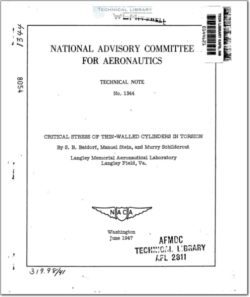17,004 Documents in our Technical Library

AA-CP-20212-001
AA-CP-20212-001
ADPO10769 Occurrence of Corrosion in Airframes
The purpose of this lecture is to provide an overview ...
MIL-STD-1759 Rivets and Rivet Type Fasteners Preferred for Design
The purpose of this book form standard is to provide ...
MIL-STD-810G Environmental Engineering Considerations and Laboratory Tests
This standard contains materiel acquisition program planning and engineering direction ...
MIL-R-7885D General Specification for Rivets, Blind, Structural, Mechanically Locked Spindle and Friction Locked Spindle
This specification covers mechanically locked spindle and  friction locked spindle ...

### NACA-TN-1344• Version
• 712.29 KB File Size
• 1 File Count
• December 4, 2015 Create Date
• December 4, 2015 Last Updated
Scroll for Details

National Advisory Committee for Aeronautics, Technical Notes - Critical Stress of Thin-Walled Cylinders in TorsionA theoretical solution is_given for the critical_stress of
thin-walled cylinders loaded in torsion} The results are presented
in terms of a few simple formulas and curves _which are applicable
to a wide range of cylinder dimensions from very short cylinders of
results are found to be in somewhat better agreement with experi—.
mental results than previous theoretical work for the same range
of cylinder dimensions.

For most practical purposes the solution to the problem of
the buckling of cylinders in torsion was given by DonneILin an
important contribution to shell theory published in 1933 (reference 1).
The present paper, which gives a solution to the same problenn
has two main objectives: first, to present a theoretical solution
of somewhat improved accuracy; second, to help complete a series
of papers treating the buckling strength ofcurved sheetfrom a
unified viewpoint based on a method .of analysis essentially
equivalent to that of Donnell but considerably simpler. (See,
for example,_references 2 and 3.)

The method of solution in the present'paper is that developed
in reference 3. The steps in the theoretical computations of the
critical stress are contained in the'appendix. The results are
given in the form of nondimensional curves and simple approximate
formulas which follow these curves closely in the usual range of
cylinder dimensions.

The values of ks for cylinders with either simply supported or
clamped edges are given in the form of logarithmic plots in
figure 1. The ordinate in this figure is the critical shear-
stress coefficient ks The abscissa is a curvature parameter Z
which is given directly by the theory and involves the dimensions
of the cylinder and Poisson s ratio.

For very short cylinders the value of the shear-stress coef-
ficient approaches the values for flat plates, 5.31!- when the edges
are simply supported and 8. 98 when the edges are clamped. As Z
increases ks also increases and the curves which defined 1:5
are given approximately by straight lines.

FileAction
naca-tn-1344.pdf

### NACA-TN-1344• Version
• 712.29 KB File Size
• 1 File Count
• December 4, 2015 Create Date
• December 4, 2015 Last Updated
Scroll for Details

National Advisory Committee for Aeronautics, Technical Notes - Critical Stress of Thin-Walled Cylinders in TorsionA theoretical solution is_given for the critical_stress of
thin-walled cylinders loaded in torsion} The results are presented
in terms of a few simple formulas and curves _which are applicable
to a wide range of cylinder dimensions from very short cylinders of
results are found to be in somewhat better agreement with experi—.
mental results than previous theoretical work for the same range
of cylinder dimensions.

For most practical purposes the solution to the problem of
the buckling of cylinders in torsion was given by DonneILin an
important contribution to shell theory published in 1933 (reference 1).
The present paper, which gives a solution to the same problenn
has two main objectives: first, to present a theoretical solution
of somewhat improved accuracy; second, to help complete a series
of papers treating the buckling strength ofcurved sheetfrom a
unified viewpoint based on a method .of analysis essentially
equivalent to that of Donnell but considerably simpler. (See,
for example,_references 2 and 3.)

The method of solution in the present'paper is that developed
in reference 3. The steps in the theoretical computations of the
critical stress are contained in the'appendix. The results are
given in the form of nondimensional curves and simple approximate
formulas which follow these curves closely in the usual range of
cylinder dimensions.

The values of ks for cylinders with either simply supported or
clamped edges are given in the form of logarithmic plots in
figure 1. The ordinate in this figure is the critical shear-
stress coefficient ks The abscissa is a curvature parameter Z
which is given directly by the theory and involves the dimensions
of the cylinder and Poisson s ratio.

For very short cylinders the value of the shear-stress coef-
ficient approaches the values for flat plates, 5.31!- when the edges
are simply supported and 8. 98 when the edges are clamped. As Z
increases ks also increases and the curves which defined 1:5
are given approximately by straight lines.

FileAction
naca-tn-1344.pdf
17,004 Documents in our Technical Library